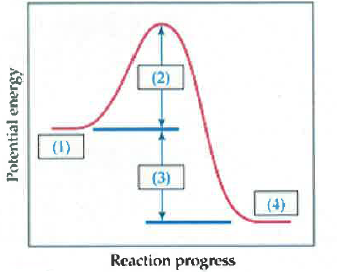Problem: For the reaction in the below diagram, explain whether the forward or reverse reaction greater in rate A. The reverse reaction because its activation energy is lower B. The reverse reaction because its activation energy is higher C. The forward reaction because its activation energy is lower D. The forward reaction because its activation energy is higher

FREE Expert Solution
82% (144 ratings)
Problem Details

For the reaction in the below diagram, explain whether the forward or reverse reaction greater in rate

A. The reverse reaction because its activation energy is lower

B. The reverse reaction because its activation energy is higher

C. The forward reaction because its activation energy is lower

D. The forward reaction because its activation energy is higherWhat scientific concept do you need to know in order to solve this problem?

Our tutors have indicated that to solve this problem you will need to apply the Energy Diagrams concept. You can view video lessons to learn Energy Diagrams Or if you need more Energy Diagrams practice, you can also practice Energy Diagrams practice problems .

What is the difficulty of this problem?

Our tutors rated the difficulty of For the reaction in the below diagram, explain whether the f... as medium difficulty.

How long does this problem take to solve?

Our expert Chemistry tutor, Sabrina took 3 minutes to solve this problem. You can follow their steps in the video explanation above.

What professor is this problem relevant for?

Based on our data, we think this problem is relevant for Professor Daoudi's class at UCF.